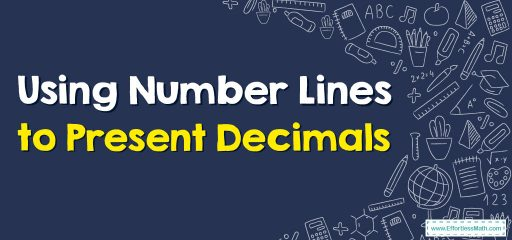# Using Number Lines to Represent DecimalsDecimals are basically used to write numbers that are not whole. These numbers are in between two whole numbers.
The numbers before the decimal point are called the whole number and all numbers after the decimal point is considered parts of a whole.
To present decimals on the number lines, each unit length between 0 and 1 is divided into 10 parts.
For instance, between 0 and 1, you get 0.1,0.2,0.3,0.4,0.5,0.6,0.7,0.7,0.8,0.9.

### Using Number Lines to Present Decimals – Examples 1

Find number 1.4 on the number line.

Solutions:

Step 1: Jump one number to reach 1.
Step 2: Divide the unit length between 1 and 2 into 10 identical parts on the number line. Each interval represents 0.1.
Step 3: Count the intervals to reach 1.4.

### Using Number Lines to Present Decimals – Examples 2

Find number 2.7 on the number line.

Solutions:

Step 1: Jump two numbers to reach 2.
Step 2: Divide the unit length between 2 and 3 into 10 identical parts on the number line. Each interval represents 0.1.
Step 3: Count the intervals to reach 2.7.

### What people say about "Using Number Lines to Represent Decimals - Effortless Math: We Help Students Learn to LOVE Mathematics"?

No one replied yet.

X
30% OFF

Limited time only!

Save Over 30%

SAVE $5 It was$16.99 now it is \$11.99